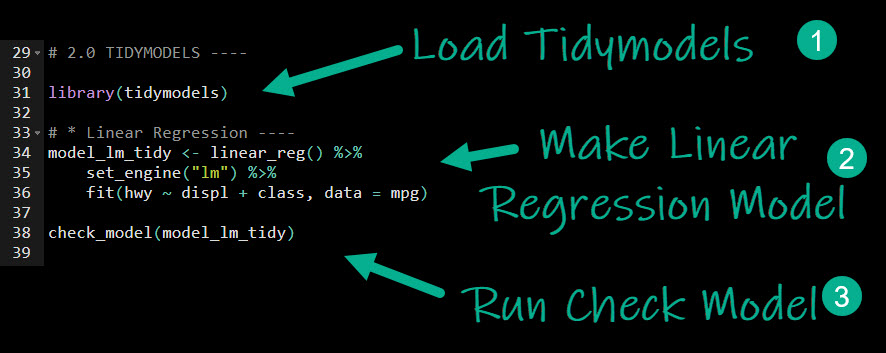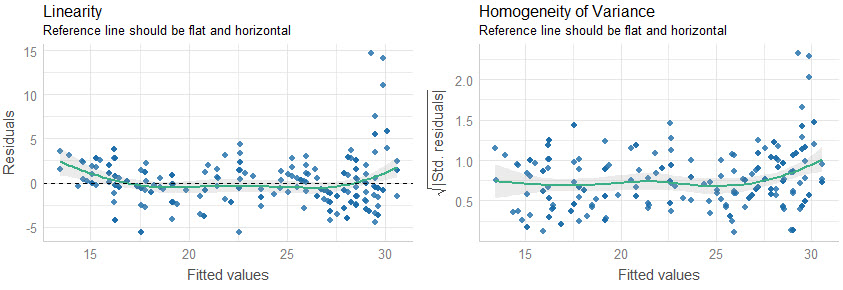Want to share your content on R-bloggers? click here if you have a blog, or here if you don't.

Easystats `performance` is an R package that makes it easy to investigate the relevant assumptions for regression models. Simply use the `check_model()` function to produce a visualization that combines 6 tests for model performance. We’ll quickly:

• Learn how to investigate performance with `performance::check_model()`
• Check out the `Tidymodels` integration with `check_model()`
• Step through each of the 6 Model Performance Plots so you know how to use them.

## R-Tips Weekly

Here are the links to get set up. ?

# Video TutorialFor those that prefer Full YouTube Video Tutorials.

Learn how to use `performance::check_model()` in our 7-minute YouTube video tutorial.

# What is Model Performance?

Model performance is the ability to understand the quality of our model predictions. This means both understanding if we have a good model and where our model is susceptible to poor predictions. We’ll see how with `performance::check_model()`.

`performance` is a new R package for evaluating statistical models in R. It provides a suite of tools to measure and evaluate model performance. We’ll focus on the `check_model()` function, which makes a helpful plot for analyzing the model quality of regression models.We’ll go through a short tutorial to get you up and running with `performance::check_model()`.

# Before we get started, get the R Cheat Sheet

`performance` is great for making quick plots of model performance. But, you’ll still need to learn how to model data with `tidymodels`. For those topics, I’ll use the Ultimate R Cheat Sheet to refer to `tidymodels` code in my workflow.

### Quick Example:Now you’re ready to quickly reference the `tidymodels` ecosystem and functions.Onto the tutorial.

# Model Performance Tutorial

Let’s get up and running with the `performance` package using `check_model()` with the `tidymodels` integration so we can assess Model Performance.

## Load the Libraries and Data

First, run this code to:

1. Load Libraries: Load `performance` and `tidyverse`.
2. Import Data: We’re using the `mpg` dataset that comes with `ggplot2`.Load the data. We’re using the `mpg` dataset.## Linear Regression: Make and Check Models

Next, we’ll quickly make a Linear Regression model with `tidymodels`. Then I’ll cover more specifics on what we are doing. Refer to the Ultimate R Cheat Sheet for more on Tidymodels beyond what we cover here. Alternatively, check out my R for Business Analysis Course (DS4B 101-R) to learn Tidymodels in-depth.

### Modeling: Making and Checking the Tidymodels Linear Regression Model

Here’s the code. We follow 3-Steps:

1. Load Tidymodels: This loads `parsnip` (the modeling package in the tidymodels ecosystem)

2. Make Linear Regression Model: We set up a model specification using `linear_reg()`. We then select an engine with `set_engine()`. In our case we want “lm”, which connects to `stats::lm()`. We then `fit()` the model. We use a formula `hwy ~ displ + class` to make highway fuel economy our target and displacement and vehicle class our predictors. This creates a trained model.

3. Run Check Model: With a fitted model in hand, we can run `performance::check_model()`, which generates the Model Performance Plot.### Model Performance Plot

Here is the output of `check_model()`, which returns a Model Performance Plot. This is actually 6-plots in one. We’ll go through them next.Let’s go through the plots, analyzing our model performance.

## Analyzing the 6 Model Performance Plots

Let’s step through the 6-plots that were returned.

### Residual Linearity

The first two plots analyze the linearity of the residuals (in-sample model error) versus the fitted values. We want to make sure that our model is error is relatively flat.Quick Assessment:

• We can see that when our model predictions are around 30, our model has larger error compared to below 30. We may want to inspect these points to see what could be contributing to the lower predictions than actuals.

## Collinearity and High Leverage Points

The next two plots analyze for collinearity and high leverage points. Collinearity is when features are highly correlated, which can throw off simple regression models (more advanced models use a concept called regularization and hyperparameter tuning to control for collinearity). High Leverage Points are observations that deviate far from the average. These can skew the predictions for linear models, and removal or model adjustment may be necessary to control model performance.Quick Assessment:

• Collinearity: We can see that both of the features have low collinearity (green bars). No model adjustments are necessary.

• Influential Observations: None of the predictins are outside of the contour lines indicating we don’t have high leverage points. No model adjustments are necessary.

## Normality of Residuals

The last two plots analyze for the normality of residuals, which is how the model error is distributed. If the distributions are skewed, this can indicate problems with the model.Quick Assessment:

• Quantile-Quantile Plot: We can see that several points towards the end of the quantile plot do fall along the straight-line. This indicates that the model is not predicting well for these points. Further inspection is required.

• Normal Density Plot: We can see there is a slight increase in density around 15, which looks to shift the distribution to the left of zero. This means that the high-error predictions should be investigated further to see why the model is far off on this subset of the data.

# Summary

We learned how to use the `check_model()` function from the `performance` package, which makes it easy to quickly analyze regression models for model performance. But, there’s a lot more to modeling.

It’s critical to learn how to build predictive models with `tidymodels`, which is the premier framework for modeling and machine learning in R.

If you’d like to learn `tidymodels` and data science for business, then read on. ?

# My Struggles with Learning Data Science

It took me a long time to learn data science. And I made a lot of mistakes as I fumbled through learning R. I specifically had a tough time navigating the ever increasing landscape of tools and packages, trying to pick between R and Python, and getting lost along the way.

If you feel like this, you’re not alone.

In fact, that’s the driving reason that I created Business Science and Business Science University (You can read about my personal journey here).

What I found out is that:

1. Data Science does not have to be difficult, it just has to be taught smartly

2. Anyone can learn data science fast provided they are motivated.

# How I can help

If you are interested in learning R and the ecosystem of tools at a deeper level, then I have a streamlined program that will get you past your struggles and improve your career in the process.

It’s called the 5-Course R-Track System. It’s an integrated system containing 5 courses that work together on a learning path. Through 5+ projects, you learn everything you need to help your organization: from data science foundations, to advanced machine learning, to web applications and deployment.

The result is that you break through previous struggles, learning from my experience & our community of 2000+ data scientists that are ready to help you succeed.

Ready to take the next step? Then let’s get started.

## ? Top R-Tips Tutorials you might like:

Want these tips every week? Join R-Tips Weekly.